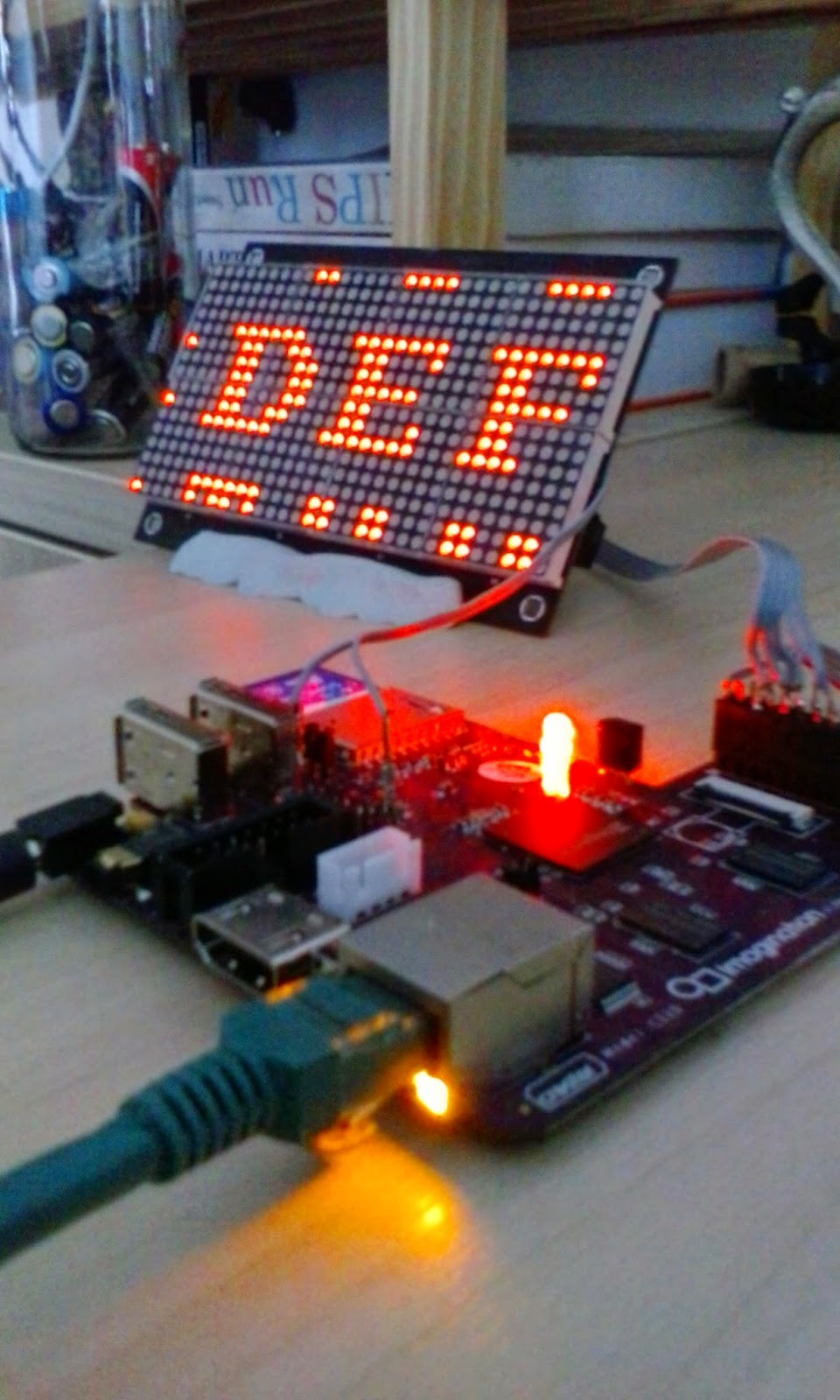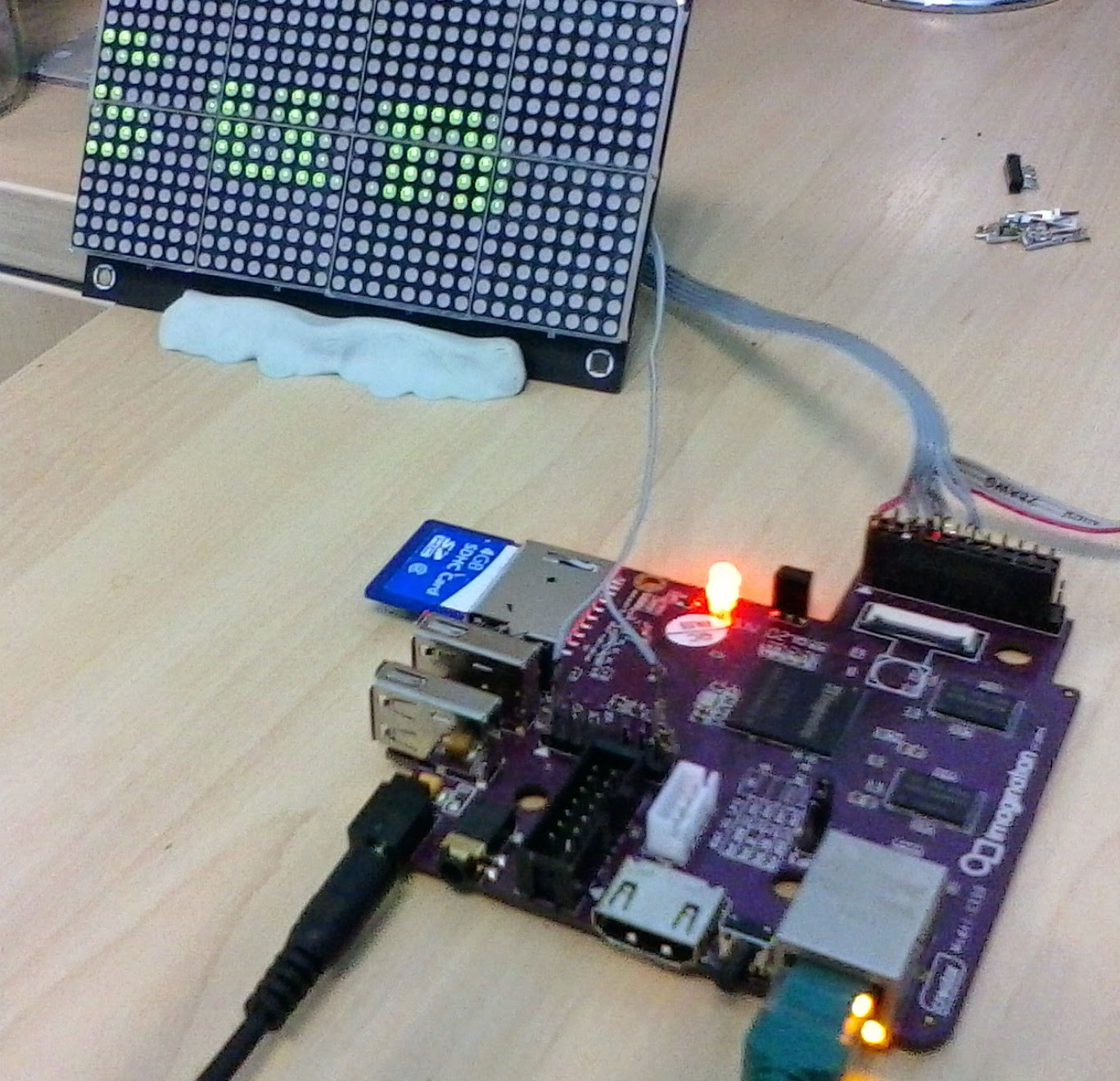## Sunday, 12 April 2015

### Printing On the Panel

This is actually pretty simple, given the work to date. It could be a lot faster, but for simple scrolling messages then this should be plenty fast enough.

To plot out bitmaps we take three steps:
• Generate a main bitmap
• Break down the four grid regions of each panel (one per HT1632C)
• Render each grid region bitmap

Doing this in reverse (bottom up)

### A single HT1632C Renderer

We can define a single handler for a 16 * 8 LED grid, controlled by a single HT1632C chip, as:
#define DISPLAY_W (16)
#define DISPLAY_H (8)
#define DISPLAY_SZ (DISPLAY_W*DISPLAY_H)

class Grid
{

public:
Grid();
virtual ~Grid();
...

private:
char _pixels_green[DISPLAY_SZ];
char _pixels_red[DISPLAY_SZ];
DisplayInterface* _interface;
int _display_chip;
};
Where _pixels_green[] and _pixels_red[] are simply bitmaps for the LED states. We can set a single pixel value with
void Grid::SetPixel(int x, int y, char value_g, char value_r)
{
int offset;

if ((x >= DISPLAY_W) || (y>= DISPLAY_H))
{
printf("Display data set is out of range\n");
return;
}
offset = (y * DISPLAY_W) + x;
_pixels_green[offset] = value_g;
_pixels_red[offset] = value_r;
}

and the writeout for green looks something like:
reg = 0;
dcursor = 0;
for (int x = 0 ; x < DISPLAY_W; x++)
{
for (int y=0; y < DISPLAY_H; y++)
{
int offset = (y * DISPLAY_W) + x;

if (_pixels_green[offset] != 0)
{
reg |=1;
}
else
{
reg &=0xfe;
}
if ((dcursor+1) %4 == 0)
{
_interface->Command(_display_chip, 0x5, dcursor/4, reg);
}
else
{
reg = reg <<1;
}
dcursor++;
}
}

This loads the registers for each column from the incoming bitmap, writing out the register value every four pixels. X=Y=0 is in the top left hand corner of the display in this case. Other than this the class is simply getters and setters for the chip select on this panel (_display_chip) and the appropriate hardware interface controller, as well as grid clear operations (just memset the pixel arrays).

The red LED writeout operates using the same logic, only 32 registers higher.

### A Four Grid (Panel) Renderer

This is  actually very simple - we create a master "bitmap" class for our main 32 * 16 display and it aggregates the grid class above:

#define BITMAP_WIDTH (32)
#define BITMAP_HEIGHT (16)
#define BITMAP_SZ (BITMAP_WIDTH*BITMAP_HEIGHT)

class BitMapper
{

public:
BitMapper();
virtual ~BitMapper();
...
private:
char _pixels_green[BITMAP_SZ];
char _pixels_red[BITMAP_SZ];

Grid _grid;
DisplayInterface _display;
};

This has an almost identical setup logic for pixels as the Grid code from earlier. So now we can load and write out a single bitmap to the underlying panels with logic like:

_grid.SetPanel(0);
_grid.ClearPixels();
for (x=0; x <16; x++)
{
for (y=0; y < 8; y++)
{
int offset = (y * BITMAP_WIDTH) + x;
_grid.SetPixel(x, y, _pixels_green[offset], _pixels_red[offset]);
}
}
_grid.Writeout();

_grid.SetPanel(1);
_grid.ClearPixels();
for (x=16; x <32; x++)
for (y=0; y < 8; y++)
{
int offset = (y * BITMAP_WIDTH) + x;

_grid.SetPixel(x-16, y, _pixels_green[offset], _pixels_red[offset]);
}

_grid.Writeout();

and so on for for all four panels
i.e. we're simply looping over sections of the source bitmap, and setting the appropriate sub-panel select and pixel value.

### The top level renderer

This is also fairly trivial - we create a pixel map for rendering characters and simply drop this into the BitMapper data, then invoke a write and flush it to the display. There's a discussion of bitmap fonts here and I'll just grab the Font Image here as an example of font grid comprising of 10*10 characters.

To get this into our source code we can just use The Gimp and load up the image, then autocrop it (to ensure the characters are on a grid from 0,0) and export as "C Source Code".

This produces the image data as a simple C-Style array:

/*
* GIMP RGB C-Source image dump (rexpaint_cp437_10x10.c)
* From http://www.gridsagegames.com/blog/2014/09/font-creation/
*/

#define GIMP_IMAGE_WIDTH (160)
#define GIMP_IMAGE_HEIGHT (160)
#define GIMP_IMAGE_BYTES_PER_PIXEL (3) /* 3:RGB, 4:RGBA */
#define GIMP_IMAGE_PIXEL_DATA ((unsigned char*) GIMP_IMAGE_pixel_data)

static const unsigned char GIMP_IMAGE_pixel_data[160 * 160 * 3 + 1] =
("\0\0\0\0\0\0\0\0\0\0\0\0\0\0\0\0\0\0\0\0\0\0\0\0\0\0\0\0\0\0\0\0\0\0\0\0\377"
...

In this code we have defines for the image width, height and pixel depth, as well as the image data array so we can reference a single pixel with code such as:
void GimpImport::SampleMap(int x, int y, char* red, char* green)
{
int index;

index = y*GIMP_IMAGE_WIDTH*GIMP_IMAGE_BYTES_PER_PIXEL;
index += x*GIMP_IMAGE_BYTES_PER_PIXEL;

*red = GIMP_IMAGE_pixel_data[index++];
*green = GIMP_IMAGE_pixel_data[index++];
return;
}

And we can copy single character glyphs out of the source bitmap and into a destination bitmap:

void GimpImport::RenderGlyph(int which, int x, int y)
{
int xin, yin;

xin = ((which*_glyph_w) % GIMP_IMAGE_WIDTH);
yin = (which/_glyph_per_line)* _glyph_h;

for (int ln =0; ln < _glyph_h; ln++)
{
for (int xg =0; xg < _glyph_w; xg++)
{
char g,r;
SampleMap(xin+xg, yin, &r, &g);
PutPixel(x+xg, y, r, g);
}
y++;
yin++;
}
}

By combining this with a couple of simple strings we can then simply dump to the output panel:Section of Character MapScrolling date text